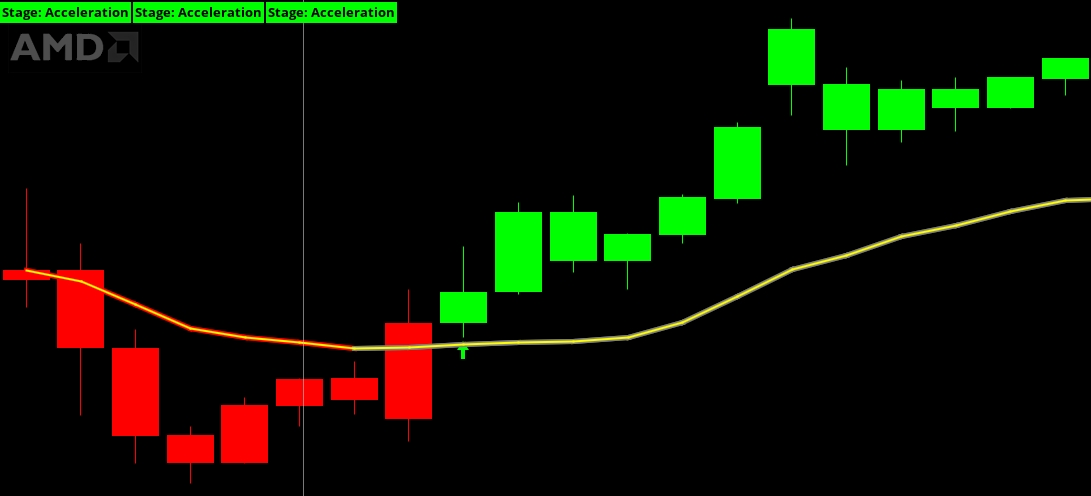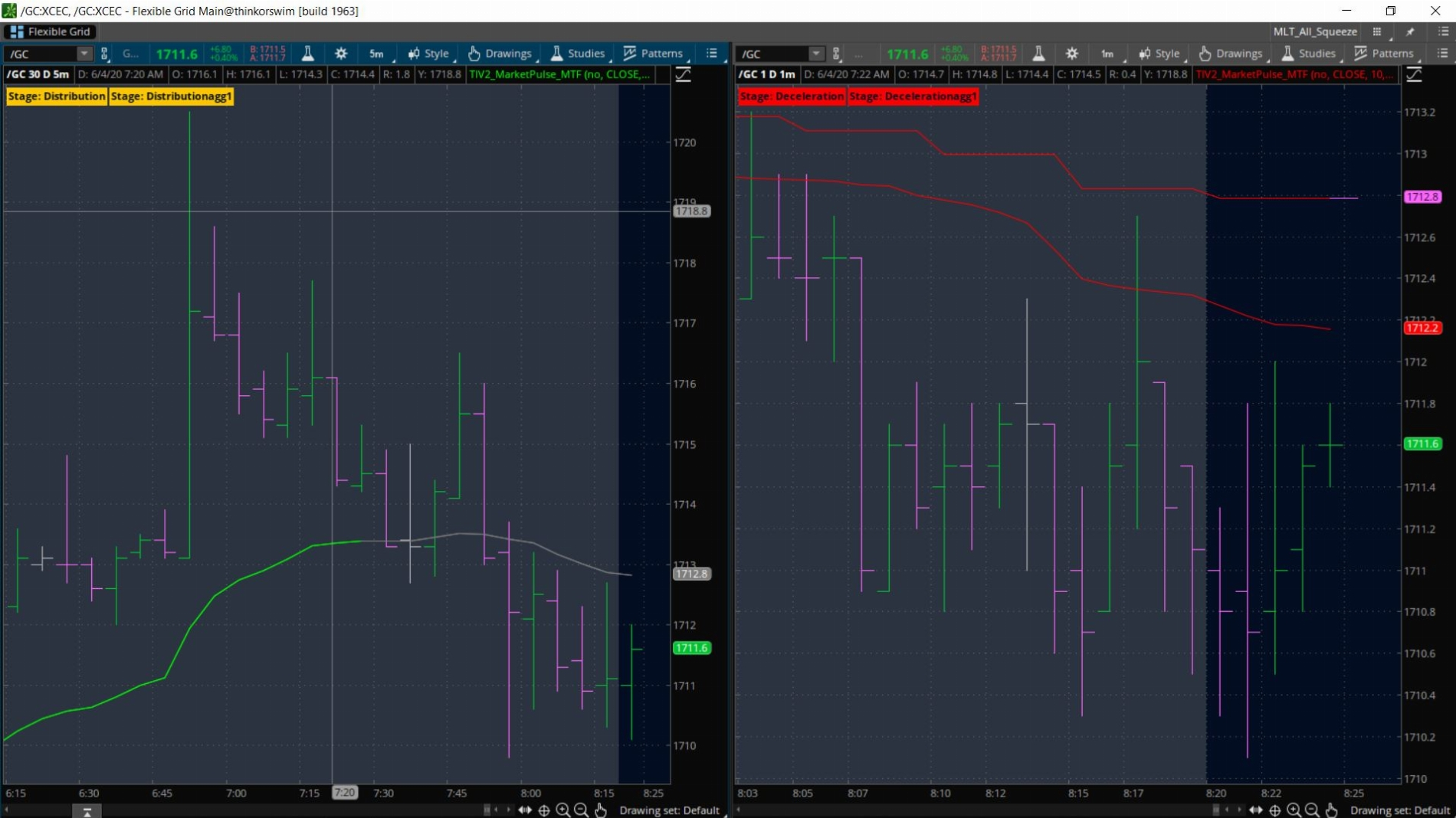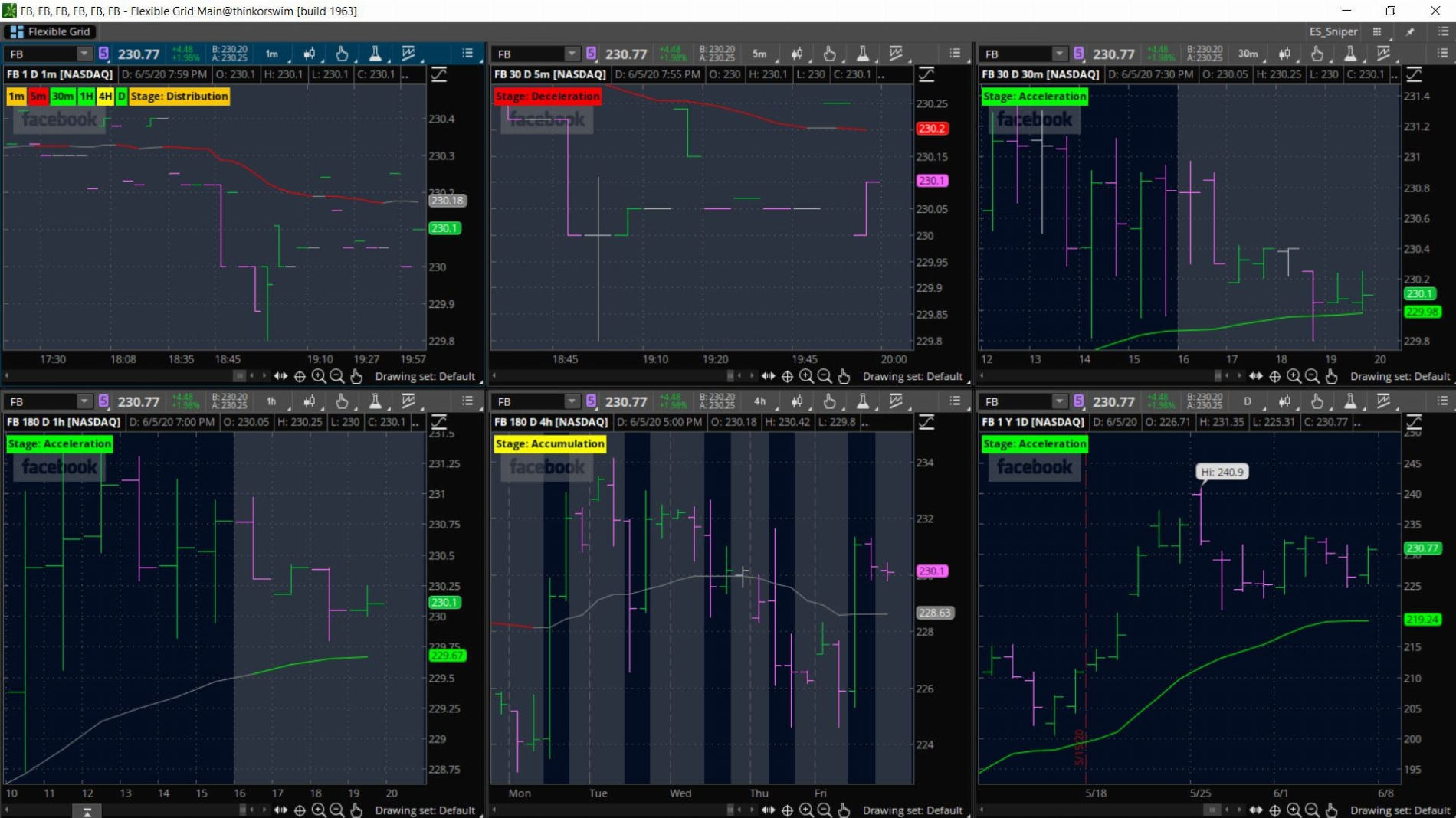# TI_MarketPulse Scan, Label, Alerts, MTF

#### shanc

##### New member
Hi members, I have this free indicator from https://tosindicators.com/ called MarketPulse. I would appreciate it if anyone could add the MultiTimeFrame feature to this Indicator. I tried combining it myself using other MTF scripts but it's not working. Thank you for your time and if anyone is interested they have a bunch of other useful and free indicators on their site as well. Good day.
Code:
``````#TOS Indicators
#Home of the Volatility Box
#Code written in 2019

input ChartBubblesOn = no;

input price = close;
input length = 10;

def tmp1 = if price > price then price - price else 0;
def tmp2 = if price > price then price - price else 0;
def d2 = sum(tmp1, length);
def d4 = sum(tmp2, length);
def cond = d2 + d4 == 0;
def ad3 = if cond then 0 else (d2 - d4) / (d2 + d4) * 100;
def coeff = 2 / (length + 1) * AbsValue(ad3) / 100;
def asd = compoundValue("visible data" = coeff * price + (if IsNaN(asd) then 0 else asd) * (1 - coeff), "historical data" = price
);
plot VMA = asd;
VMA.setDefaultColor(GetColor(0));

def vwma8 = sum(volume * close, 8) / sum(volume, 8);
def vwma21 = sum(volume * close, 21) / sum(volume, 21);
def vwma34 = sum(volume * close, 34) / sum(volume, 34);

def bullish = if vwma8 > vwma21 and vwma21 > vwma34 then 1 else 0;
def bearish = if vwma8 < vwma21 and vwma21 < vwma34 then 1 else 0;
def distribution = if !bullish and !bearish then 1 else 0;

AddLabel(yes, if bullish then "Stage: Acceleration" else if bearish then "Stage: Deceleration" else if close>=VMA then "Stage: Accumulation" else "Stage: Distribution", if bullish then color.green else if bearish then color.red else if close >=VMA then color.yellow else color.orange);

VMA.AssignValueColor(if bearish and close<= VMA then color.red
else if bullish and close >= VMA then color.green
else color.gray);

def accumulationToAcceleration = if (bullish and close>=VMA) then 1 else 0;
AddChartBubble(ChartBubblesOn and accumulationToAcceleration and !accumulationToAcceleration, close, "Entering Acceleration", color.green);

def distributionToDeceleration = if (bearish and close <= VMA) then 1 else 0;
AddChartBubble(ChartBubblesOn and distributionToDeceleration and !distributionToDeceleration, close, "Entering Deceleration", color.red);``````

#### horserider

##### Well-known member
VIP
Code:
``````#TOS Indicators
#Home of the Volatility Box
#Code written in 2019
# Second aggregation period added by horserider 5/31/2020

input ChartBubblesOn = no;

input price = close;
input length = 10;

def tmp1 = if price > price then price - price else 0;
def tmp2 = if price > price then price - price else 0;
def d2 = sum(tmp1, length);
def d4 = sum(tmp2, length);
def cond = d2 + d4 == 0;
def ad3 = if cond then 0 else (d2 - d4) / (d2 + d4) * 100;
def coeff = 2 / (length + 1) * AbsValue(ad3) / 100;
def asd = compoundValue("visible data" = coeff * price + (if IsNaN(asd) then 0 else asd) * (1 - coeff), "historical data" = price
);
plot VMA = asd;
VMA.setDefaultColor(GetColor(0));

def vwma8 = sum(volume * close, 8) / sum(volume, 8);
def vwma21 = sum(volume * close, 21) / sum(volume, 21);
def vwma34 = sum(volume * close, 34) / sum(volume, 34);

def bullish = if vwma8 > vwma21 and vwma21 > vwma34 then 1 else 0;
def bearish = if vwma8 < vwma21 and vwma21 < vwma34 then 1 else 0;
def distribution = if !bullish and !bearish then 1 else 0;

AddLabel(yes, if bullish then "Stage: Acceleration" else if bearish then "Stage: Deceleration" else if close>=VMA then "Stage: Accumulation" else "Stage: Distribution", if bullish then color.green else if bearish then color.red else if close >=VMA then color.yellow else color.orange);

VMA.AssignValueColor(if bearish and close<= VMA then color.red
else if bullish and close >= VMA then color.green
else color.gray);

def accumulationToAcceleration = if (bullish and close>=VMA) then 1 else 0;
AddChartBubble(ChartBubblesOn and accumulationToAcceleration and !accumulationToAcceleration, close, "Entering Acceleration", color.green);

def distributionToDeceleration = if (bearish and close <= VMA) then 1 else 0;
AddChartBubble(ChartBubblesOn and distributionToDeceleration and !distributionToDeceleration, close, "Entering Deceleration", color.red);

######### Second aggregation
input agg1 = aggregationPeriod.DAY;

def tmp1agg1 = if close(period = Agg1) > close(period = Agg1) then close(period = Agg1) - close(period = Agg1) else 0;
def tmp2agg1 = if close(period = Agg1) > close(period = Agg1) then close(period = Agg1) - close(period = Agg1) else 0;

def d2agg1 = sum(tmp1agg1, length);
def d4agg1 = sum(tmp2agg1, length);
def condagg1 = d2agg1 + d4agg1 == 0;
def ad3agg1 = if condagg1 then 0 else (d2agg1 - d4agg1) / (d2agg1 + d4agg1) * 100;
def coeffagg1 = 2 / (length + 1) * AbsValue(ad3agg1) / 100;
def asdagg1 = compoundValue("visible data" = coeffagg1 * price + (if IsNaN(asdagg1) then 0 else asdagg1) * (1 - coeffagg1), "historical data" = price
);
plot VMAagg1 = asdagg1;
VMAagg1.setDefaultColor(GetColor(0));

def vwma8agg1 = sum(volume(period = Agg1) * close(period = Agg1), 8) / sum(volume(period = Agg1), 8);
def vwma21agg1 = sum(volume(period = Agg1) * close(period = Agg1), 21) / sum(volume(period = Agg1), 21);
def vwma34agg1 = sum(volume(period = Agg1) * close(period = Agg1), 34) / sum(volume(period = Agg1), 34);

def bullishagg1 = if vwma8agg1 > vwma21agg1 and vwma21agg1 > vwma34agg1 then 1 else 0;
def bearishagg1 = if vwma8agg1 < vwma21agg1 and vwma21agg1 < vwma34agg1 then 1 else 0;
def distributionagg1 = if !bullishagg1 and !bearishagg1 then 1 else 0;

AddLabel(yes, if bullishagg1 then "Stage: Acceleration" else if bearishagg1 then "Stage: Decelerationagg1" else if close(period = Agg1)>=VMAagg1 then "Stage: Accumulationagg1" else "Stage: Distributionagg1", if bullishagg1 then color.green else if bearishagg1 then color.red else if close(period = Agg1) >=VMAagg1 then color.yellow else color.orange);

VMAagg1.AssignValueColor(if bearishagg1 and close(period = Agg1)<= VMAagg1 then color.red
else if bullishagg1 and close(period = Agg1) >= VMAagg1 then color.green
else color.gray);

def accumulationToAccelerationagg1 = if (bullishagg1 and close(period = Agg1)>=VMAagg1) then 1 else 0;
AddChartBubble(ChartBubblesOn and accumulationToAccelerationagg1 and !accumulationToAccelerationagg1, close(period = Agg1), "Entering Accelerationagg1", color.green);

def distributionToDecelerationagg1 = if (bearishagg1 and close(period = Agg1) <= VMAagg1) then 1 else 0;
AddChartBubble(ChartBubblesOn and distributionToDecelerationagg1 and !distributionToDecelerationagg1, close(period = Agg1), "Entering Decelerationagg1", color.red);``````

Last edited:
•shanc and wtf_dude

#### shanc

##### New member
Dear @horserider, thank you for your kind work. I've tried the script but it does not match the higher time frame. Attached the screenshot. The chart on the right is the original script on 1H and the left is the new script on 5M and aggregation 1H.Also, I was originally looking for something of a corner label with the aggregation period colored. The plotted line would only serve as an added advantage. It was my mistake for not being clear on my request and I apologize for the inconvenience. Once again, thank you @horserider.•sindhu19

#### horserider

##### Well-known member
VIP
Put this study on 1 hour chart with an agg of 1 hour. Added the original but colored in yellow. Looks like all align.•wtf_dude

#### shanc

##### New member
Thank you for your reply. Can you guide me on how it can show the higher time frame plot on a lower time frame?. Good day to you.

#### jay2

##### Member
why the multiple labels?

#### horserider

##### Well-known member
VIP
@shanc If your aggregation is set to say 1 hour , just choose any time frame of less than 1 hour for your chart.

@jay2 Chart in post # 4 has multiple labels because of the studies on the chart. First there is the muti-agg study, so it will show 2 labels. One for the chart time frame and one for your aggregation time frame. The third one is from the original study added to compare if time frames plotted correctly.

•jay2

#### shanc

##### New memberHye @horserider, what I meant was it doesn't show the change of color on the plot or label. The price does match the Higher Time Frame. On the 1M chart, I've set it to 5M Agg. For the higher time frame plot, it should be showing a gray line and Stage: Distributionagg1 instead it's following the current time frame. For sharing your knowledge and taking the time to help, I thank you @horserider. Good day.

#### horserider

##### Well-known member
VIP
@shanc Ahh I changed the code. May have had some errors. The new code is in post #2. Check if that works as it should. Sorry if I messed up.
@wtf_dude @jay2

•shanc

#### shanc

##### New member
I know I've said it a few times but once again thank you @horserider. Very kind of you to offer your guidance. The script works beautifully.
With everyone's selfless contribution to this site, I'm inspired as well. Below is the script with MTF Labels added to MarketPulse Script. To the one seeking this script, I don't know how to code, so forgive me if something isn't right. I kinda copy-pasted from here and there..The 1M displays the labels with color for the selected timeframes, the original script is on other charts for reference.

Code:
``````#TOS Indicators
#Home of the Volatility Box
#Code written in 2019
#Second aggregation period added by horserider 5/31/2020
#Added Labels with TimeFrame & Color, removed line plot and cleaned code by ShancR 06/06/2020
#Color Stages: Green = Acceleration, Orange = Distribution, Red = Decelaration, Yellow = Accumulation

input price = close;
input length = 10;

######### Second aggregation
input agg1 = AggregationPeriod.DAY;

def tmp1agg1 = if close(period = agg1) > close(period = agg1) then close(period = agg1) - close(period = agg1) else 0;
def tmp2agg1 = if close(period = agg1) > close(period = agg1) then close(period = agg1) - close(period = agg1) else 0;

def d2agg1 = Sum(tmp1agg1, length);
def d4agg1 = Sum(tmp2agg1, length);
def condagg1 = d2agg1 + d4agg1 == 0;
def ad3agg1 = if condagg1 then 0 else (d2agg1 - d4agg1) / (d2agg1 + d4agg1) * 100;
def coeffagg1 = 2 / (length + 1) * AbsValue(ad3agg1) / 100;
def asdagg1 = CompoundValue("visible data" = coeffagg1 * price + (if IsNaN(asdagg1) then 0 else asdagg1) * (1 - coeffagg1), "historical data" = price
);
def VMAagg1 = asdagg1;

def vwma8agg1 = Sum(volume(period = agg1) * close(period = agg1), 8) / Sum(volume(period = agg1), 8);
def vwma21agg1 = Sum(volume(period = agg1) * close(period = agg1), 21) / Sum(volume(period = agg1), 21);
def vwma34agg1 = Sum(volume(period = agg1) * close(period = agg1), 34) / Sum(volume(period = agg1), 34);

def bullishagg1 = if vwma8agg1 > vwma21agg1 and vwma21agg1 > vwma34agg1 then 1 else 0;
def bearishagg1 = if vwma8agg1 < vwma21agg1 and vwma21agg1 < vwma34agg1 then 1 else 0;
def accumulationagg1 = if close(period = agg1) >= VMAagg1 then 1 else 0;
def distributionagg1 = if close(period = agg1) <= VMAagg1 then 1 else 0;

AddLabel(yes, if agg1== aggregationPeriod.MONTH then "M"
else
if agg1== aggregationPeriod.WEEK then "W"
else
if agg1== aggregationPeriod.FOUR_DAYS then "4D"
else
if agg1== aggregationPeriod.THREE_DAYS then "3D"
else
if agg1== aggregationPeriod.TWO_DAYS then "2D"
else
if agg1== aggregationPeriod.DAY then "D"
else
if agg1== aggregationPeriod.FOUR_HOURS then "4H"
else
if agg1== aggregationPeriod.TWO_HOURS then "2H"
else
if agg1== aggregationPeriod.HOUR then "1H"
else
if agg1== aggregationPeriod.THIRTY_MIN then "30m"
else
if agg1== aggregationPeriod.TWENTY_MIN then "20m"
else
if agg1== aggregationPeriod.FIFTEEN_MIN then "15m"
else
if agg1== aggregationPeriod.TEN_MIN then "10m"
else
if agg1== aggregationPeriod.FIVE_MIN then "5m"
else
if agg1== aggregationPeriod.FOUR_MIN then "4m"
else
if agg1== aggregationPeriod.THREE_MIN then "3m"
else
if agg1== aggregationPeriod.TWO_MIN then "2m"
else
if agg1== aggregationPeriod.MIN then "1m"
else "", if bullishagg1 then Color.GREEN
else if bearishagg1 then Color.RED
else if accumulationagg1 then Color.YELLOW
else if distributionagg1 then Color.ORANGE
else Color.WHITE
);``````

#### dinodotcom

##### Member
2019 Donor
Hello,

Thanks for the great script. Is there anyway to make this into a scan?

#### shanc

##### New member
Hye @dinodotcom, unfortunately not my expertise. I hope others can help with that. Good day.

#### mansor

##### Active member
I have installed script from post #10 so it would assist me in understanding what phase is the ticker in.

however, after installing and applying, I have set agg to 5m on 5m1d chart and 1m on 1m1d chart. I am getting two labels on each chart, how can I fix this to just one label? On 5m1d chart labels are exactly same, & on 1m1d chart, the first label reads "stage:acceleration" and second label reads "stage: accelerationagg1"

On 1h10D chart I have stage:acceleration and stage:decelerationagg1
the length is 10 on each time frame/chart
How can I fix this please

Last edited:

#### shanc

##### New member
@mansor If you're getting labels with "stage: acceleration" and "stage: accelerationagg1" then It's coming from Post #2 script. Post#10 script does not have these label codes. Take care.

Last edited:
•mansor

#### mansor

##### Active member
@mansor If you're getting labels with "stage: acceleration" and "stage: accelerationagg1" then It's coming from Post #2 script. Post#10 script does not have these label codes.

THANK you for correcting me, I just updated it.

•shanc

#### Necr0sis

##### New member
VIP
@shanc I've been trying to use your modification of the script with the labels, but it doesn't seem to work for me. The previous scripts work fine, but I don't see anything with yours other than a yellow box in the top left corner showing the aggregation period. Your help would be appreciated!

#### shanc

##### New member
Hi @Necr0sis, thats how it was meant to work. The updated version doesn't plot any lines. It only shows the color of the plot on agg timeframes in the box. Set the chart to the lower time frame and set a higher agg for the labels. Add as many aggs as you need. Good day.

•Necr0sis

#### Necr0sis

##### New member
VIP
Hi @Necr0sis, thats how it was meant to work. The updated version doesn't plot any lines. It only shows the color of the plot on agg timeframes in the box. Set the chart to the lower time frame and set a higher agg for the labels. Add as many aggs as you need. Good day.

The updated script in post #2 works fine, but the one you made with the labels doesn't seem to be working for me. I have a higher aggregation set in the study. I don't seem to see anything on my chart. Your help would be appreciated!

#### shanc

##### New member
By any chance, you're using the Tick or Renko bars @Necr0sis ? Because this study will not work with them as they do not have a higher time agg for reference. If the problem persists, do share a screenshot with us.

•Necr0sis

#### Necr0sis

##### New member
VIP
By any chance, you're using the Tick or Renko bars @Necr0sis ? Because this study will not work with them as they do not have a higher time agg for reference. If the problem persists, do share a screenshot with us.

I'm using standard candles.

### Not the exact question you're looking for?

87k+ Posts
197 Online## The Market Trading Game Changer

Join 2,500+ subscribers inside the useThinkScript VIP Membership Club
• Exclusive indicators
• Proven strategies & setups
• Private Discord community
• Exclusive members-only content
• 1 full year of unlimited support

What is useThinkScript?

useThinkScript is the #1 community of stock market investors using indicators and other tools to power their trading strategies. Traders of all skill levels use our forums to learn about scripting and indicators, help each other, and discover new ways to gain an edge in the markets.

How do I get started?

We get it. Our forum can be intimidating, if not overwhelming. With thousands of topics, tens of thousands of posts, our community has created an incredibly deep knowledge base for stock traders. No one can ever exhaust every resource provided on our site.

If you are new, or just looking for guidance, here are some helpful links to get you started.

What are the benefits of VIP Membership?